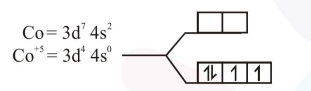# The one that can exhibit highest paramagnetic behaviour among the following is :-

Question:

The one that can exhibit highest paramagnetic behaviour among the following is :-

$\mathrm{gly}=\mathrm{glycinato} ; \mathrm{bpy}=2,2^{\prime}-$ bipyridine

1. $\left[\mathrm{Pd}(\mathrm{gly})_{2}\right]$

2. $\left[\mathrm{Ti}\left(\mathrm{NH}_{3}\right)_{6}\right]^{3+}$

3. $\left[\mathrm{Co}(\mathrm{OX})_{2}(\mathrm{OH})_{2}\right]^{-}\left(\Delta_{0}>\mathrm{P}\right)$

4. $\left[\mathrm{Fe}(\text { en })(\text { bpy })\left(\mathrm{NH}_{3}\right)_{2}\right]^{2+}$

Correct Option: , 3

Solution:

$\left[\mathrm{Co}(\mathrm{OX})_{2}(\mathrm{OH})_{2}\right]^{-} \quad \Delta_{0}>\mathrm{P} \quad[\mathrm{S} . \mathrm{F} . \mathrm{L}]$It has highest number of unpaired e-s. so it is most paramagnetic.